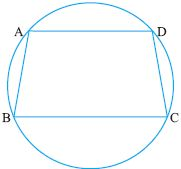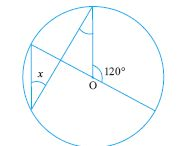Name: ___________________Date:___________________

kwizNET Subscribers, please login to turn off the Ads!
 Email us to get an instant 20% discount on highly effective K-12 Math & English kwizNET Programs!

### High School Mathematics - 28.6 Circles - Theorems

 Statement: If two arcs are congruent, then their chords are equal. Given: Arc ASB = Arc CTD To prove: AB = CDProof: OA = OlC, OB = OlD (radii) Angle AOB = Angle COlD (given that the arcs are congruent) Hence by SAS Postulate on congruence AOB is congruent to COlD Hence AB = CD Statement: There is only one circle passing through three non collinear points.Example: In the given figure prove that AB = CDSolution: Arc BAD congruent Arc CDA Hence Arc BAD - Arc AD = Arc CAD - Arc AD Arc BA is congruent Arc CD Hence AB = CD Directions: Solve the following.Name: ___________________Date:___________________

### High School Mathematics - 28.6 Circles - Theorems

 Q 1: In a circle containing the equal chords AB and CD which are on the opposite side of the centre, E. Prove that BE = DE and AE = CE where E is the point of intersection of AD and BC.Answer: Q 2: Find the missing angle.55o35o30o Q 3: D is the midpoint of side BC of isosceles triangle ABC with AB = AC. Prove that the circle drawn witrh either of the equal sides as diameter pass through D.Answer: Q 4: BC is a chord of circle with centre O. A is a point on the major arc BC as shown, prove that angBAC + angOBC = 90.Answer: Q 5: Two circles intersect each other at points A and B, if AP and AQ be the respective diameters of the circle prove that PBQ is a line.Answer: Q 6: Find angle x35o55o90o Question 7: This question is available to subscribers only! Question 8: This question is available to subscribers only!

#### Subscription to kwizNET Learning System costs less than \$1 per month & offers the following benefits:

• Unrestricted access to grade appropriate lessons, quizzes, & printable worksheets
• Instant scoring of online quizzes
• Progress tracking and award certificates to keep your student motivated
• Unlimited practice with auto-generated 'WIZ MATH' quizzes
• Child-friendly website with no advertisements

© 2003-2007 kwizNET Learning System LLC. All rights reserved. This material may not be reproduced, displayed, modified or distributed without the express prior written permission of the copyright holder. For permission, contact info@kwizNET.com
For unlimited printable worksheets & more, go to http://www.kwizNET.com.